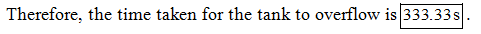# Water (ρ = 1000 kg/m3) enters a 2.00 m3 tank at a rate of 6.00 kg/s and is withdrawn at a rate of 3.00 kg/s. The tank is initially half-full. (a) Is the process continuous, batch, or semi-batch? (b) Is it transient or steady state? (c) Use the general material balance equation to determine how long it will take for the tank to overflow.

Question-AnswerCategory: Material And Energy BalanceWater (ρ = 1000 kg/m3) enters a 2.00 m3 tank at a rate of 6.00 kg/s and is withdrawn at a rate of 3.00 kg/s. The tank is initially half-full. (a) Is the process continuous, batch, or semi-batch? (b) Is it transient or steady state? (c) Use the general material balance equation to determine how long it will take for the tank to overflow.

Water enters a 2.00-m3 tank at a rate of 6.00 kg/s and is withdrawn at a rate of 3.00 kg/s. The tank is initially half full.
(a) Is this process continuous, batch, or semi-batch? Is it transient or steady state?
(b) Write a mass balance for the process. Identify the terms of the general balance equation present in your equation and state the reason for omitting any terms.
(c) How long will the tank take to overflow?